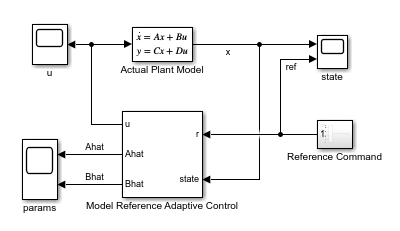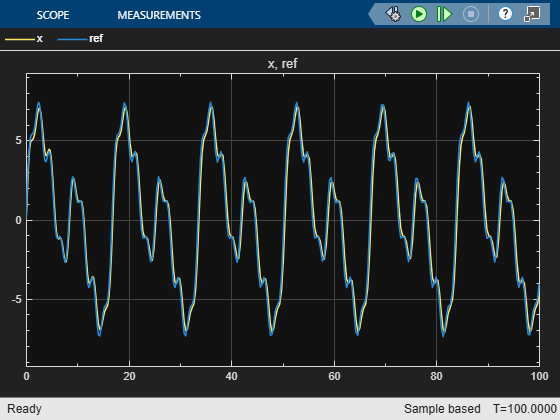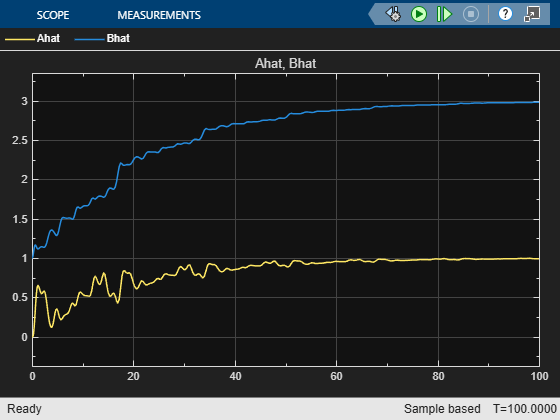# Indirect Model Reference Adaptive Control of First-Order System

This example shows how to design an indirect model reference adaptive control (MRAC) system for reference tracking. The plant is an unknown first-order system. The indirect MRAC controller estimates the plant parameters and implements an inversion-based controller to track a reference model.

### First-Order Unknown Plant Model

The controlled plant is the following first-order dynamic system.

`$\underset{}{\overset{˙}{x}}=ax\left(t\right)+bu\left(t\right)$`

Here:

• $\mathit{x}$ is the system state.

• $\mathit{a}$ and $\mathit{b}$ are the unknown system parameters.

Given this unknown nonlinear system, the goal is to design a controller that enables the tracking of the following reference model.

`${\underset{}{\overset{˙}{x}}}_{m}={a}_{m}{x}_{m}\left(t\right)+{b}_{m}r\left(t\right)$`

Here:

• ${\mathit{x}}_{\mathit{m}}$ is the reference model state.

• $\mathit{r}\left(\mathit{t}\right)$ is the reference signal provided by the user.

• ${\mathit{a}}_{\mathit{m}}$ and ${\mathit{b}}_{\mathit{m}}$ are the reference model parameters.

### Reference-Tracking Controller

The indirect MRAC controller uses an estimator model to compute $\underset{}{\overset{ˆ}{a}}$ and $\underset{}{\overset{ˆ}{b}}$, which are estimates of the unknown system parameters $\mathit{a}$ and $\mathit{b}$, respectively.

`$\underset{}{\overset{˙}{\underset{}{\overset{ˆ}{x}}}}=\underset{}{\overset{ˆ}{a}}x\left(t\right)+\underset{}{\overset{ˆ}{b}}u\left(t\right)$`

To compute the control action $\mathit{u}\left(\mathit{t}\right)$, the controller uses the feedforward gain ${\mathit{k}}_{\mathit{r}}$ and feedback gain ${\mathit{k}}_{\mathit{x}}$.

`$u\left(t\right)=-{k}_{x}x\left(t\right)+{k}_{r}r\left(t\right)$`

The controller gains are derived from the reference model parameters (${\mathit{a}}_{\mathit{m}}$ and ${\mathit{b}}_{\mathit{m}}$) and estimated observer parameters ($\underset{}{\overset{ˆ}{a}}$ and $\underset{}{\overset{ˆ}{b}}$).

`$\begin{array}{l}{k}_{r}=\frac{{b}_{m}}{\underset{}{\overset{ˆ}{b}}}\\ {k}_{x}=\frac{1}{\underset{}{\overset{ˆ}{b}}}\left({a}_{m}-\underset{}{\overset{ˆ}{a}}\right)\end{array}$`

### Configure Controller

For this example, the true reference model is as follows.

`$\underset{}{\overset{˙}{x}}=x\left(t\right)+3u\left(t\right)$`

Specify the reference model parameters, assuming that the model output corresponds to state $\mathit{x}$.

```a = 1; b = 3; c = 1; d = 0;```

This true model is unknown to the indirect MRAC controller. Instead, the controller uses an estimator model to estimate the unknown plant dynamics. During operation, the controller can adapt the parameters of this model to improve its estimate of the unknown system parameters.

Specify the initial estimator parameters.

```ahat = 0; bhat = 1;```

The goal of the controller is to track the performance of the reference model. Specify the parameters of the reference model.

```am = -4; bm = 4;```

Specify the initial condition of the plant.

`x_0 = 0;`

Specify the learning rates for updating the estimator model parameters.

```gamma_a = 2; % ahat learning rate gamma_b = 2; % bhat learning rate```

### Simulate Controller

```mdl = 'mracFirstOrder'; open_system(mdl)```In this model

• The Actual Plant Model block implements the nominal model of the first-order unknown system.

• The Reference Command block generates a reference signal.

• The Model Reference Adaptive Control block outputs the control action u, which it derives from the using the estimator model.

While an MRAC controller can also estimate unknown disturbances in the controlled system, for this example there are no such disturbances. Instead, the goal of the controller is simply to estimate the parameters of the unknown plant model. For an example that estimates unknown disturbances using a direct MRAC controller, see Model Reference Adaptive Control of Aircraft Undergoing Wing Rock.

Simulate the model.

`sim(mdl);`

View the actual plant output and the reference signal. The controller is able to make the actual plant state track the reference signal closely.

`open_system(mdl+"/state")`The Model reference Adaptive Controller block is configured to output the parameters $\underset{}{\overset{ˆ}{a}}$ and $\underset{}{\overset{ˆ}{b}}$ of the estimator model using the `Ahat` and `Bhat` output ports, respectively.

`open_system(mdl+"/params")`Over time the controller adapts the values of the estimator parameters. With sufficient persistency of excitation in the reference signal, $\underset{}{\overset{ˆ}{a}}$ and $\underset{}{\overset{ˆ}{b}}$ converge to their true values of 1 and 3, respectively.